# Frank Solutions for Class 10 Maths Chapter 9 Ratio and Proportion

Frank Solutions for Class 10 Maths Chapter 9 Ratio and Proportion are given here. Our specialists will guide you in many methods to solve a variety of questions and manage time in the examination. We therefore suggest students refer to Frank Solutions for Class 10 Maths to obtain more marks in the exam.

Chapter 9 – Ratio and Proportion contains questions related to the ratio in simplest form and comparison of ratios. The ratio of two quantities of the same kind and in the same units is the fraction that one quantity is of others. The ratio ‘a’ is to ‘b’ is the fraction (a/b), written as a : b. Some of the important topics covered in this chapter are, ratio, proportion, compound ratio, duplicate ratio, triplicate ratio, sub – duplicate ratio, sub – triplicate ratio and reciprocal ratio, etc.

## Download the PDF of Frank Solutions for Class 10 Maths Chapter 9 Ratio and Proportion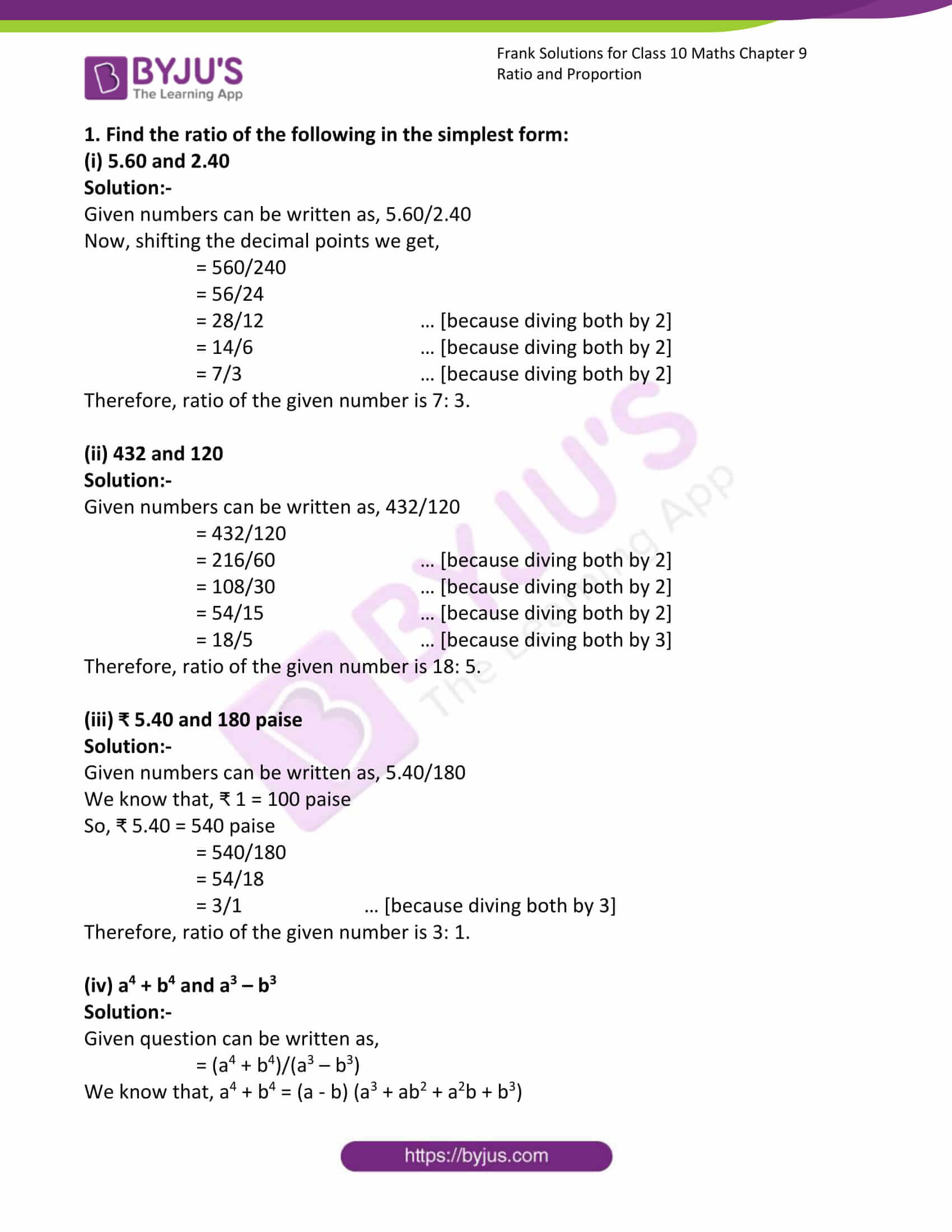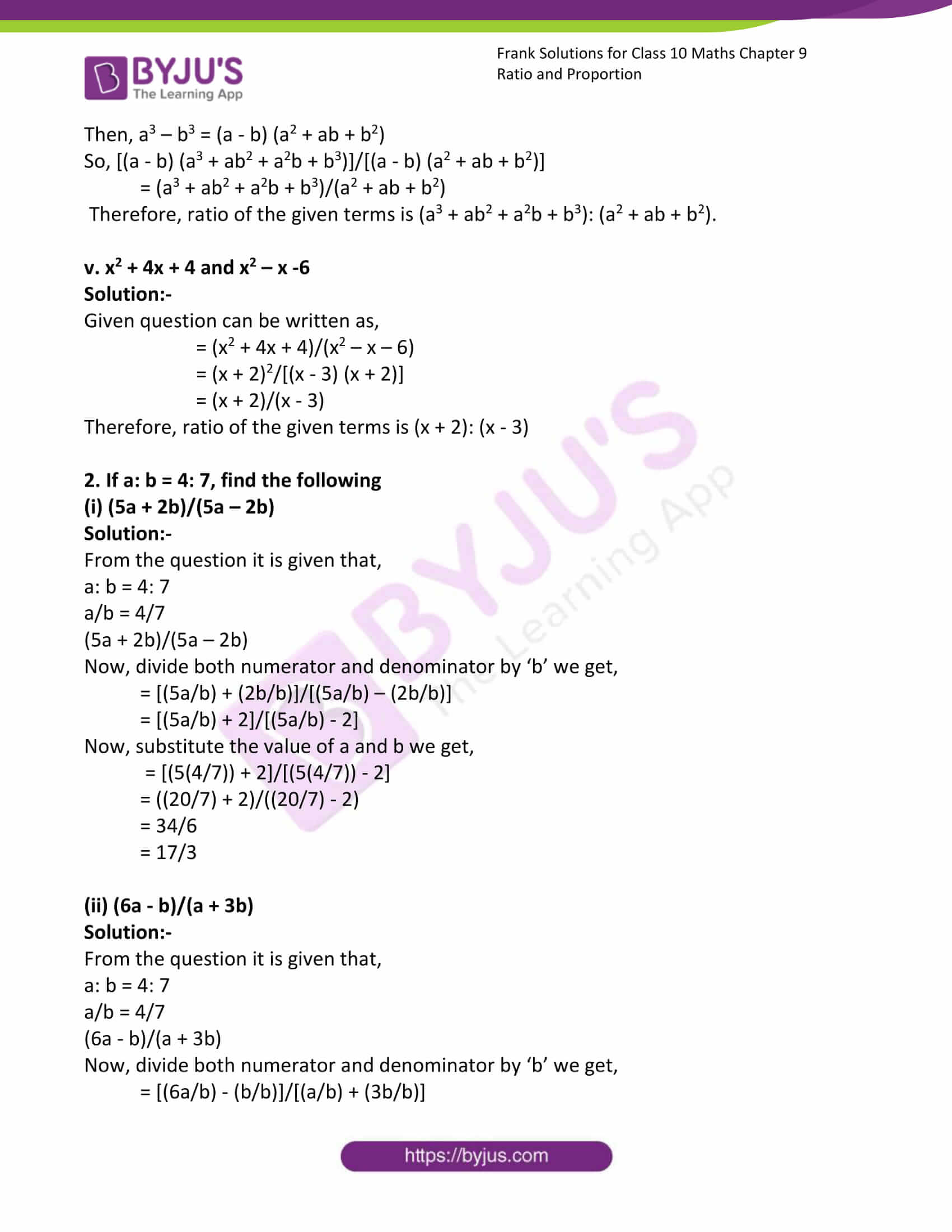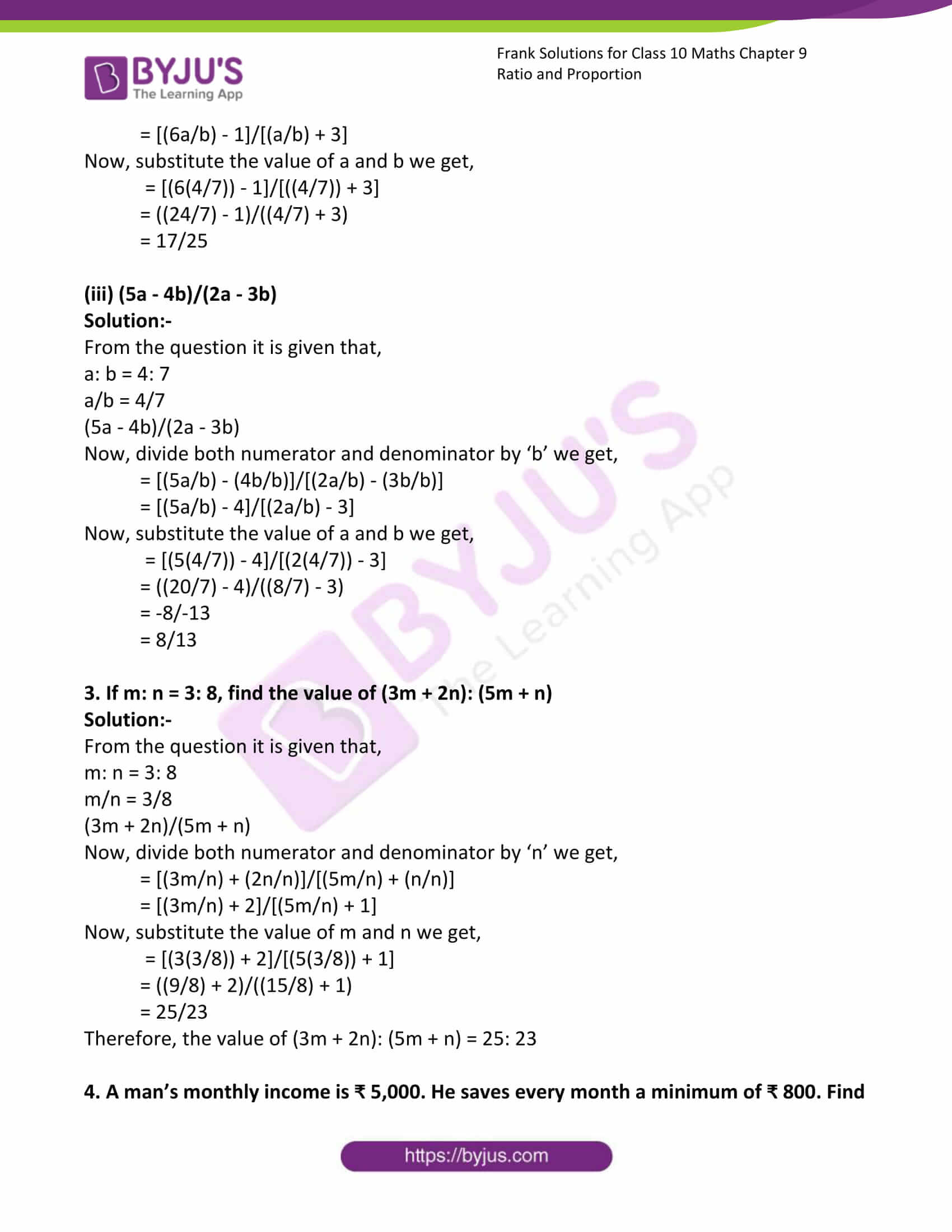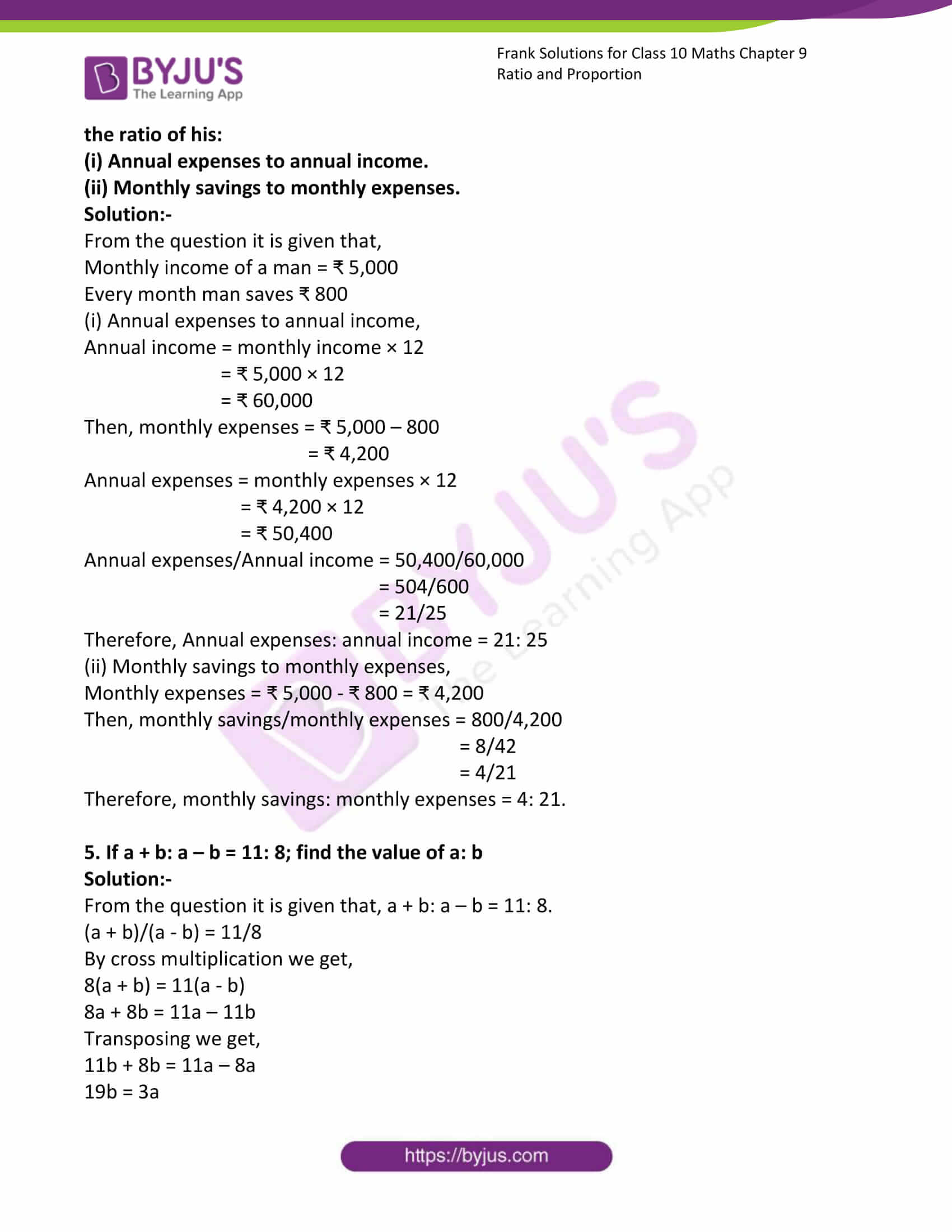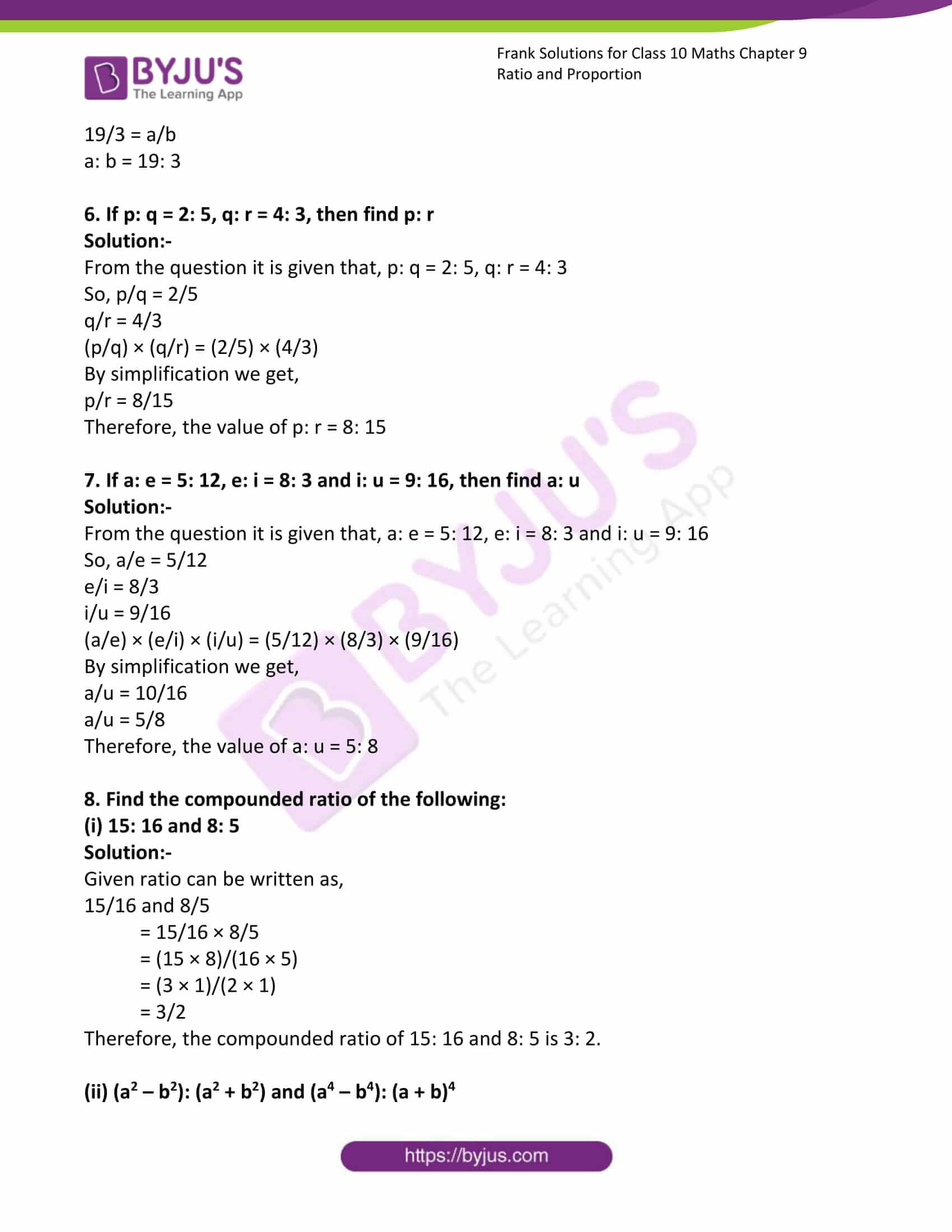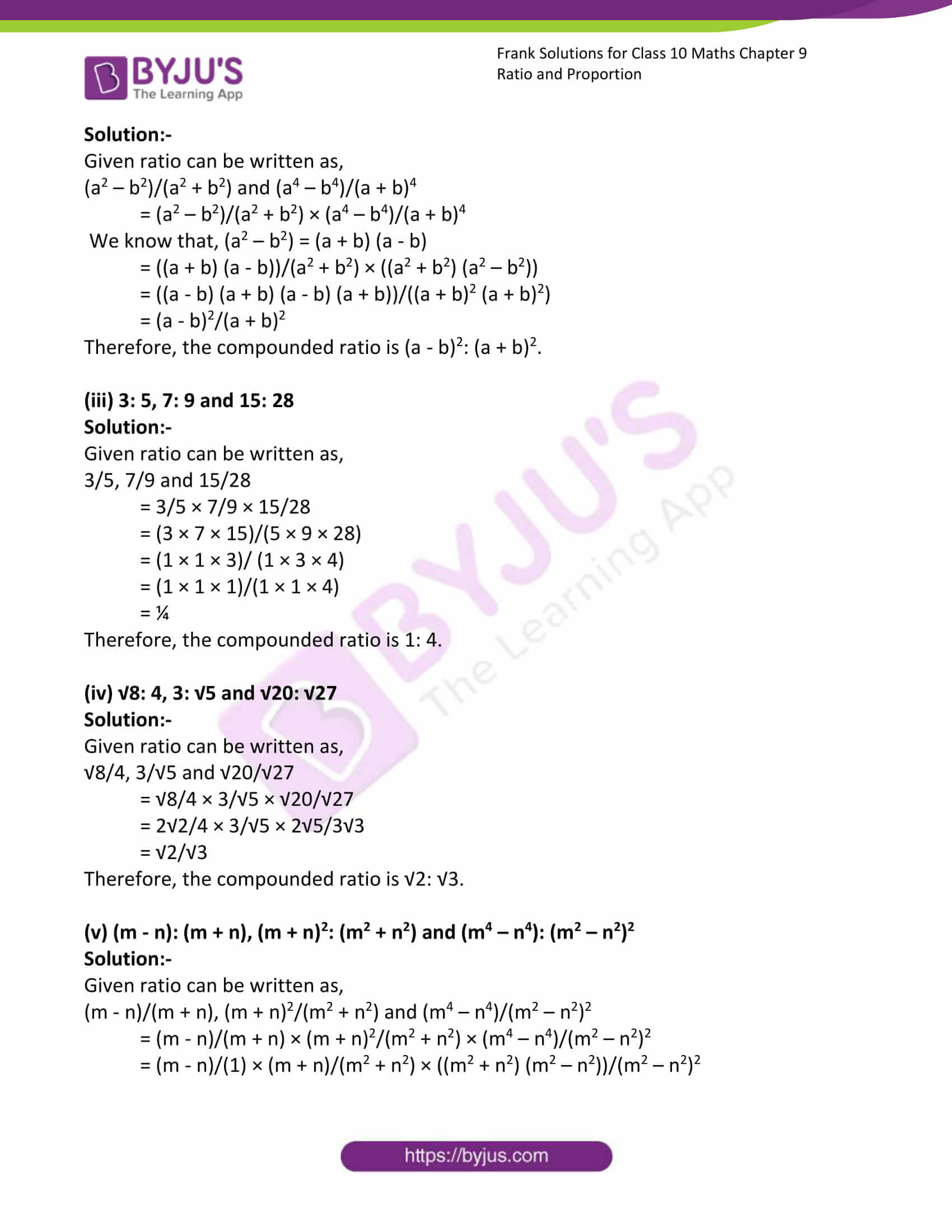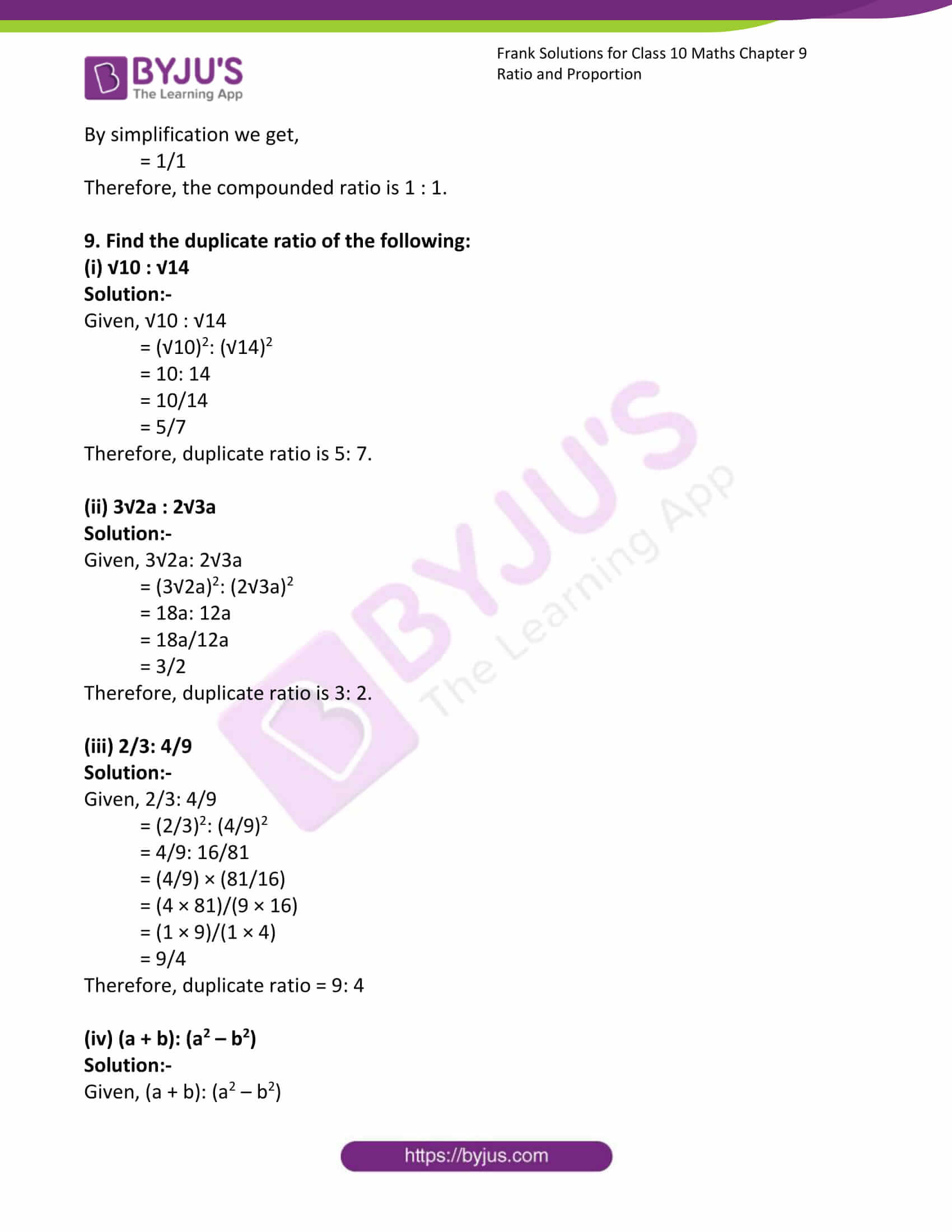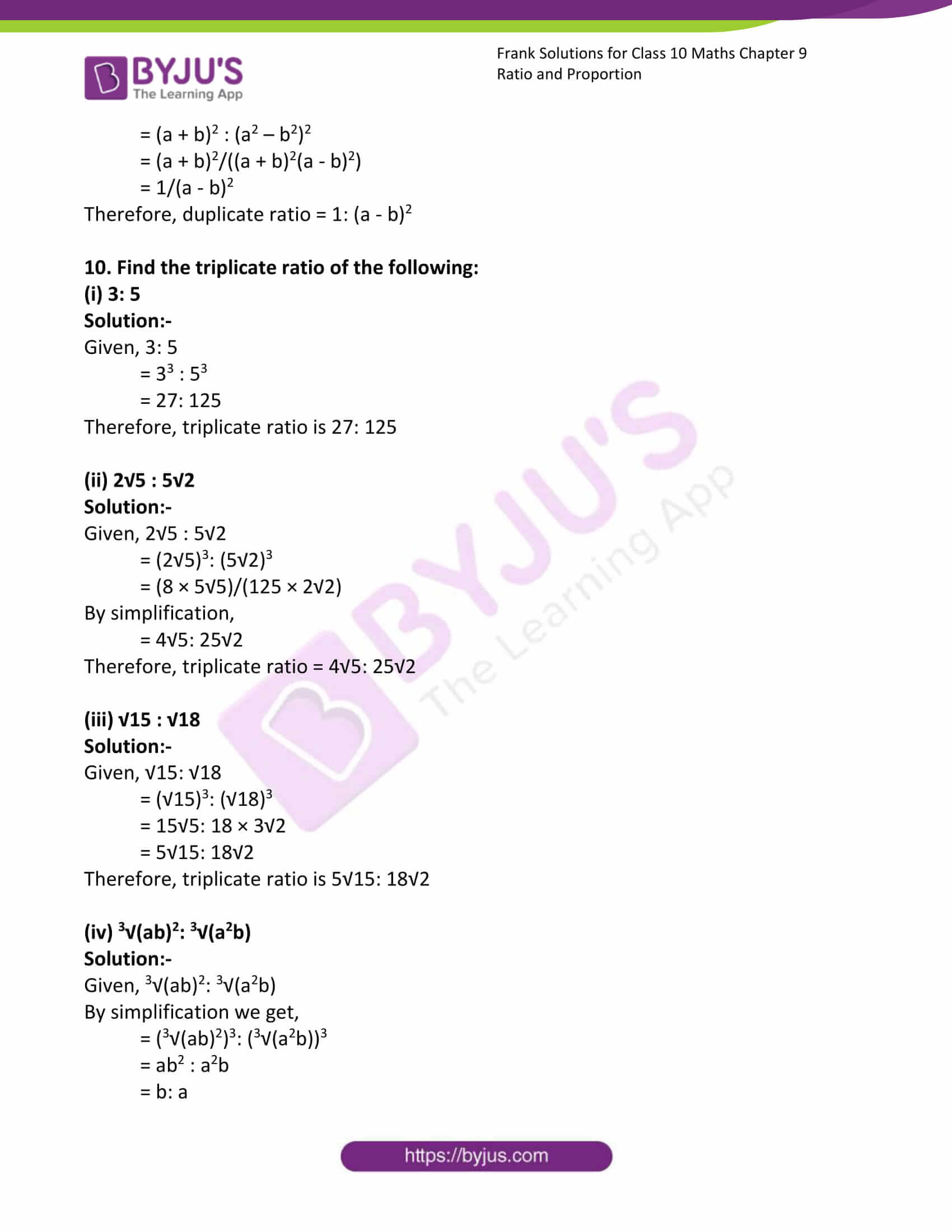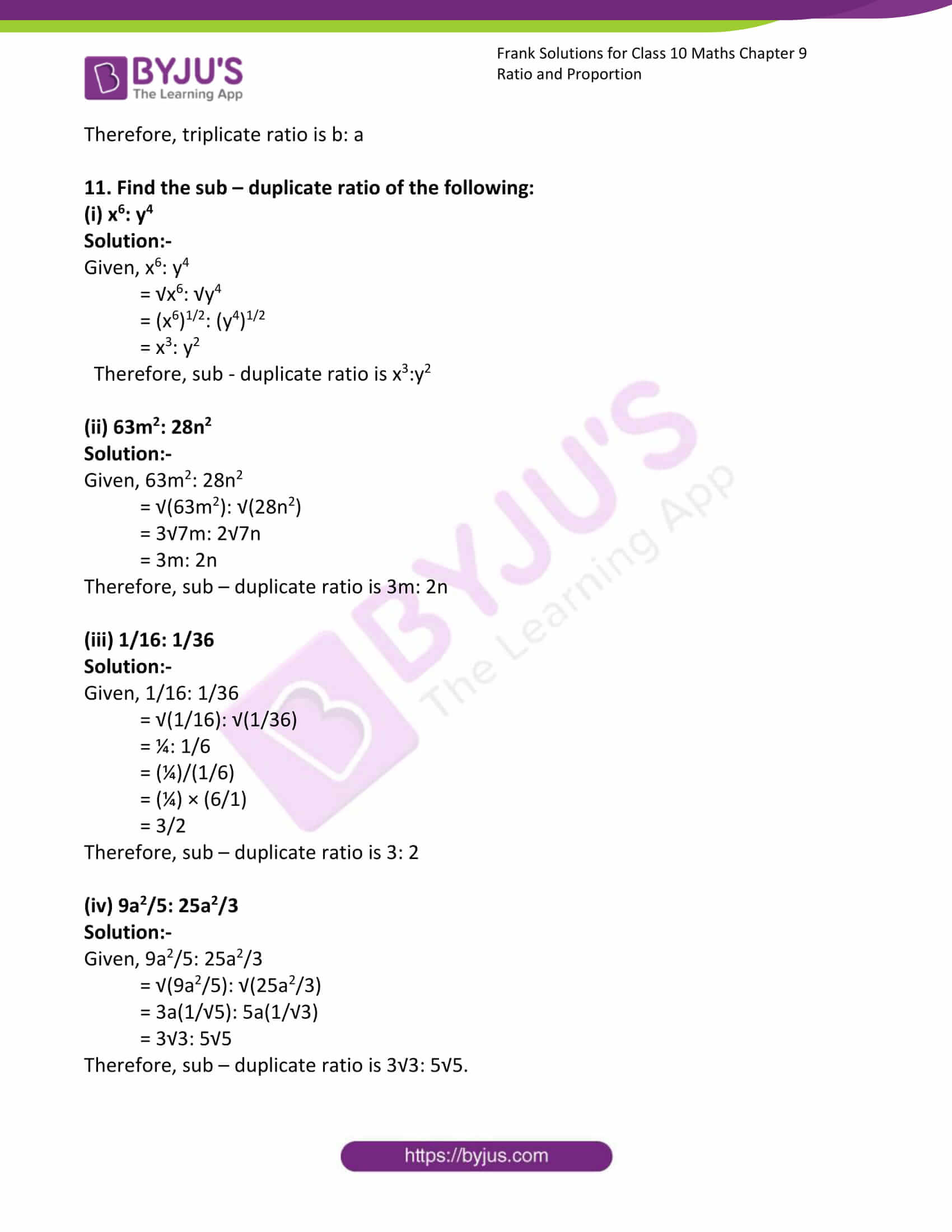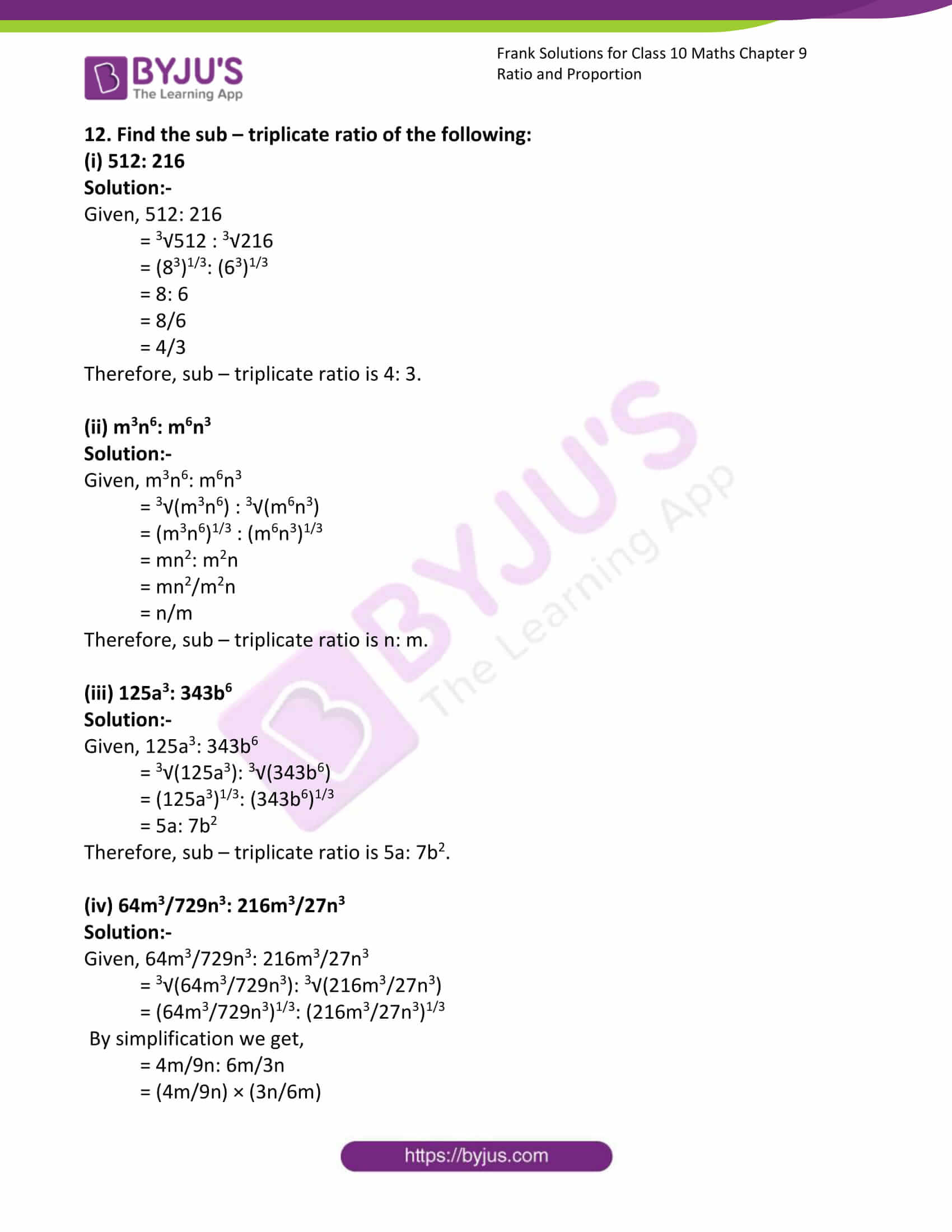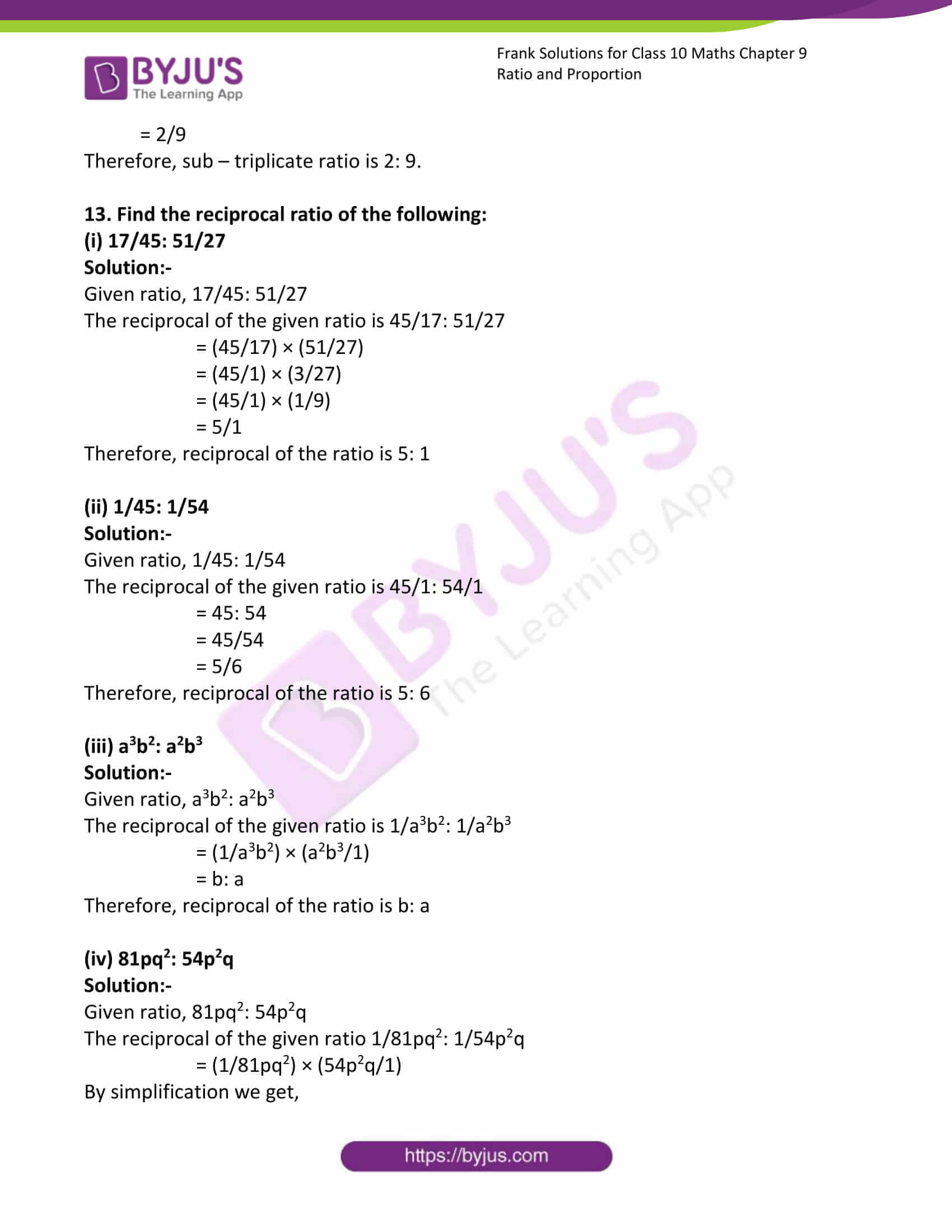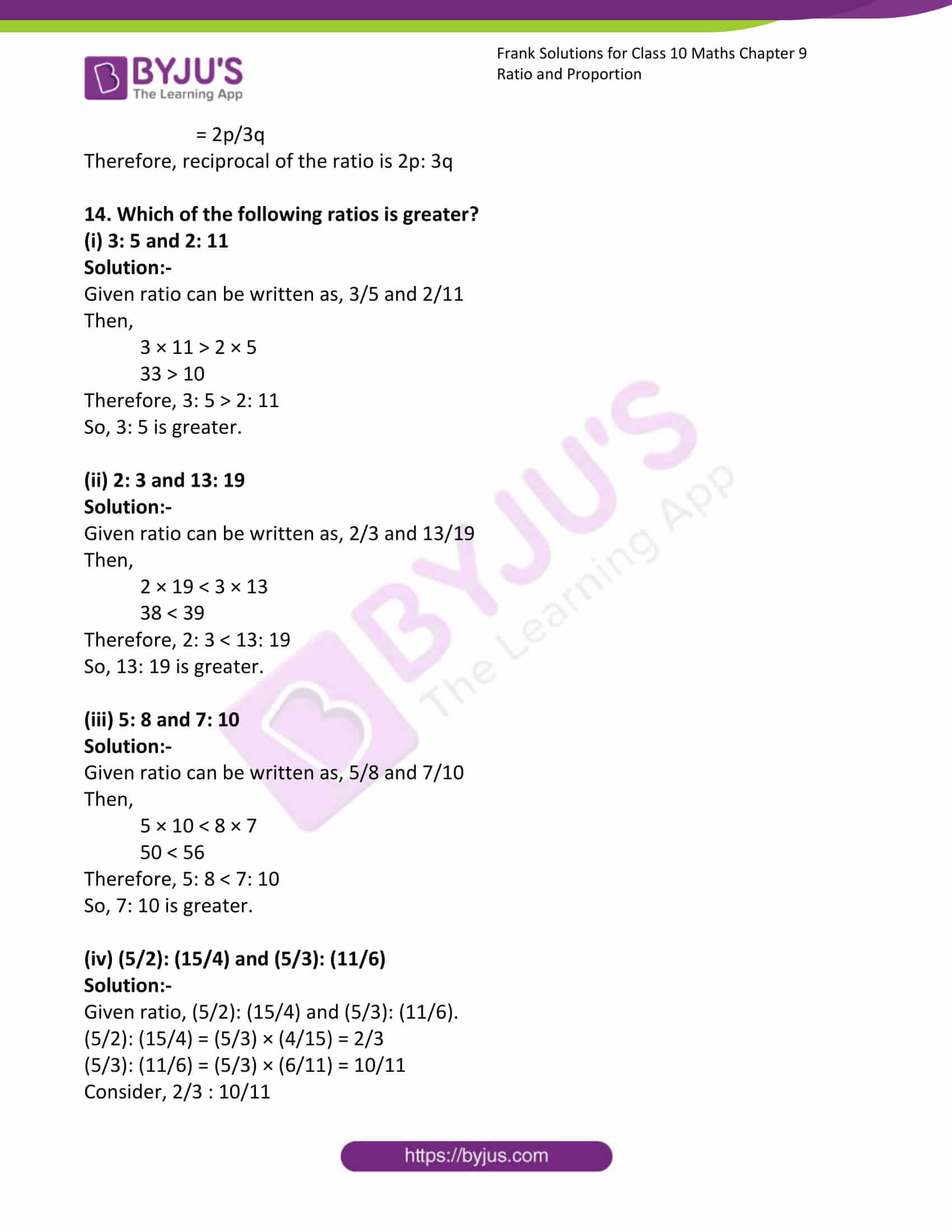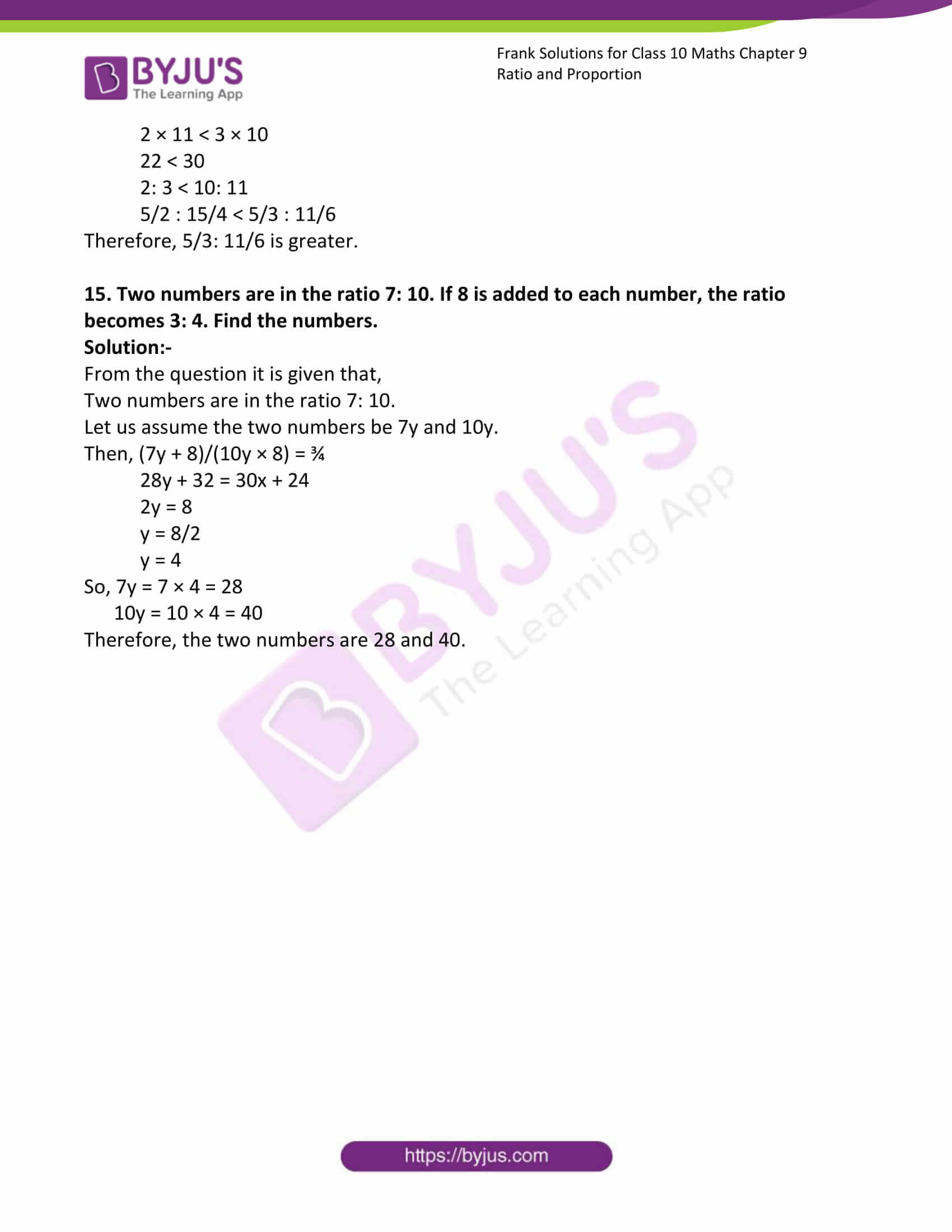### Access answers to Frank Solutions for Class 10 Maths Chapter 9 Ratio and Proportion

1. Find the ratio of the following in the simplest form:

(i) 5.60 and 2.40

Solution:-

Given numbers can be written as, 5.60/2.40

Now, shifting the decimal points we get,

= 560/240

= 56/24

= 28/12 … [because diving both by 2]

= 14/6 … [because diving both by 2]

= 7/3 … [because diving both by 2]

Therefore, ratio of the given number is 7: 3.

(ii) 432 and 120

Solution:-

Given numbers can be written as, 432/120

= 432/120

= 216/60 … [because diving both by 2]

= 108/30 … [because diving both by 2]

= 54/15 … [because diving both by 2]

= 18/5 … [because diving both by 3]

Therefore, ratio of the given number is 18: 5.

(iii) ₹ 5.40 and 180 paise

Solution:-

Given numbers can be written as, 5.40/180

We know that, ₹ 1 = 100 paise

So, ₹ 5.40 = 540 paise

= 540/180

= 54/18

= 3/1 … [because diving both by 3]

Therefore, ratio of the given number is 3: 1.

(iv) a4 + b4 and a3 – b3

Solution:-

Given question can be written as,

= (a4 + b4)/(a3 – b3)

We know that, a4 + b4 = (a – b) (a3 + ab2 + a2b + b3)

Then, a3 – b3 = (a – b) (a2 + ab + b2)

So, [(a – b) (a3 + ab2 + a2b + b3)]/[(a – b) (a2 + ab + b2)]

= (a3 + ab2 + a2b + b3)/(a2 + ab + b2)

Therefore, ratio of the given terms is (a3 + ab2 + a2b + b3): (a2 + ab + b2).

v. x2 + 4x + 4 and x2 – x -6

Solution:-

Given question can be written as,

= (x2 + 4x + 4)/(x2 – x – 6)

= (x + 2)2/[(x – 3) (x + 2)]

= (x + 2)/(x – 3)

Therefore, ratio of the given terms is (x + 2): (x – 3)

2. If a: b = 4: 7, find the following

(i) (5a + 2b)/(5a – 2b)

Solution:-

From the question it is given that,

a: b = 4: 7

a/b = 4/7

(5a + 2b)/(5a – 2b)

Now, divide both numerator and denominator by ‘b’ we get,

= [(5a/b) + (2b/b)]/[(5a/b) – (2b/b)]

= [(5a/b) + 2]/[(5a/b) – 2]

Now, substitute the value of a and b we get,

= [(5(4/7)) + 2]/[(5(4/7)) – 2]

= ((20/7) + 2)/((20/7) – 2)

= 34/6

= 17/3

(ii) (6a – b)/(a + 3b)

Solution:-

From the question it is given that,

a: b = 4: 7

a/b = 4/7

(6a – b)/(a + 3b)

Now, divide both numerator and denominator by ‘b’ we get,

= [(6a/b) – (b/b)]/[(a/b) + (3b/b)]

= [(6a/b) – 1]/[(a/b) + 3]

Now, substitute the value of a and b we get,

= [(6(4/7)) – 1]/[((4/7)) + 3]

= ((24/7) – 1)/((4/7) + 3)

= 17/25

(iii) (5a – 4b)/(2a – 3b)

Solution:-

From the question it is given that,

a: b = 4: 7

a/b = 4/7

(5a – 4b)/(2a – 3b)

Now, divide both numerator and denominator by ‘b’ we get,

= [(5a/b) – (4b/b)]/[(2a/b) – (3b/b)]

= [(5a/b) – 4]/[(2a/b) – 3]

Now, substitute the value of a and b we get,

= [(5(4/7)) – 4]/[(2(4/7)) – 3]

= ((20/7) – 4)/((8/7) – 3)

= -8/-13

= 8/13

3. If m: n = 3: 8, find the value of (3m + 2n): (5m + n)

Solution:-

From the question it is given that,

m: n = 3: 8

m/n = 3/8

(3m + 2n)/(5m + n)

Now, divide both numerator and denominator by ‘n’ we get,

= [(3m/n) + (2n/n)]/[(5m/n) + (n/n)]

= [(3m/n) + 2]/[(5m/n) + 1]

Now, substitute the value of m and n we get,

= [(3(3/8)) + 2]/[(5(3/8)) + 1]

= ((9/8) + 2)/((15/8) + 1)

= 25/23

Therefore, the value of (3m + 2n): (5m + n) = 25: 23

4. A man’s monthly income is ₹ 5,000. He saves every month a minimum of ₹ 800. Find the ratio of his:

(i) Annual expenses to annual income.

(ii) Monthly savings to monthly expenses.

Solution:-

From the question it is given that,

Monthly income of a man = ₹ 5,000

Every month man saves ₹ 800

(i) Annual expenses to annual income,

Annual income = monthly income × 12

= ₹ 5,000 × 12

= ₹ 60,000

Then, monthly expenses = ₹ 5,000 – 800

= ₹ 4,200

Annual expenses = monthly expenses × 12

= ₹ 4,200 × 12

= ₹ 50,400

Annual expenses/Annual income = 50,400/60,000

= 504/600

= 21/25

Therefore, Annual expenses: annual income = 21: 25

(ii) Monthly savings to monthly expenses,

Monthly expenses = ₹ 5,000 – ₹ 800 = ₹ 4,200

Then, monthly savings/monthly expenses = 800/4,200

= 8/42

= 4/21

Therefore, monthly savings: monthly expenses = 4: 21.

5. If a + b: a – b = 11: 8; find the value of a: b

Solution:-

From the question it is given that, a + b: a – b = 11: 8.

(a + b)/(a – b) = 11/8

By cross multiplication we get,

8(a + b) = 11(a – b)

8a + 8b = 11a – 11b

Transposing we get,

11b + 8b = 11a – 8a

19b = 3a

19/3 = a/b

a: b = 19: 3

6. If p: q = 2: 5, q: r = 4: 3, then find p: r

Solution:-

From the question it is given that, p: q = 2: 5, q: r = 4: 3

So, p/q = 2/5

q/r = 4/3

(p/q) × (q/r) = (2/5) × (4/3)

By simplification we get,

p/r = 8/15

Therefore, the value of p: r = 8: 15

7. If a: e = 5: 12, e: i = 8: 3 and i: u = 9: 16, then find a: u

Solution:-

From the question it is given that, a: e = 5: 12, e: i = 8: 3 and i: u = 9: 16

So, a/e = 5/12

e/i = 8/3

i/u = 9/16

(a/e) × (e/i) × (i/u) = (5/12) × (8/3) × (9/16)

By simplification we get,

a/u = 10/16

a/u = 5/8

Therefore, the value of a: u = 5: 8

8. Find the compounded ratio of the following:

(i) 15: 16 and 8: 5

Solution:-

Given ratio can be written as,

15/16 and 8/5

= 15/16 × 8/5

= (15 × 8)/(16 × 5)

= (3 × 1)/(2 × 1)

= 3/2

Therefore, the compounded ratio of 15: 16 and 8: 5 is 3: 2.

(ii) (a2 – b2): (a2 + b2) and (a4 – b4): (a + b)4

Solution:-

Given ratio can be written as,

(a2 – b2)/(a2 + b2) and (a4 – b4)/(a + b)4

= (a2 – b2)/(a2 + b2) × (a4 – b4)/(a + b)4

We know that, (a2 – b2) = (a + b) (a – b)

= ((a + b) (a – b))/(a2 + b2) × ((a2 + b2) (a2 – b2))

= ((a – b) (a + b) (a – b) (a + b))/((a + b)2 (a + b)2)

= (a – b)2/(a + b)2

Therefore, the compounded ratio is (a – b)2: (a + b)2.

(iii) 3: 5, 7: 9 and 15: 28

Solution:-

Given ratio can be written as,

3/5, 7/9 and 15/28

= 3/5 × 7/9 × 15/28

= (3 × 7 × 15)/(5 × 9 × 28)

= (1 × 1 × 3)/ (1 × 3 × 4)

= (1 × 1 × 1)/(1 × 1 × 4)

= ¼

Therefore, the compounded ratio is 1: 4.

(iv) √8: 4, 3: √5 and √20: √27

Solution:-

Given ratio can be written as,

√8/4, 3/√5 and √20/√27

= √8/4 × 3/√5 × √20/√27

= 2√2/4 × 3/√5 × 2√5/3√3

= √2/√3

Therefore, the compounded ratio is √2: √3.

(v) (m – n): (m + n), (m + n)2: (m2 + n2) and (m4 – n4): (m2 – n2)2

Solution:-

Given ratio can be written as,

(m – n)/(m + n), (m + n)2/(m2 + n2) and (m4 – n4)/(m2 – n2)2

= (m – n)/(m + n) × (m + n)2/(m2 + n2) × (m4 – n4)/(m2 – n2)2

= (m – n)/(1) × (m + n)/(m2 + n2) × ((m2 + n2) (m2 – n2))/(m2 – n2)2

By simplification we get,

= 1/1

Therefore, the compounded ratio is 1 : 1.

9. Find the duplicate ratio of the following:

(i) √10 : √14

Solution:-

Given, √10 : √14

= (√10)2: (√14)2

= 10: 14

= 10/14

= 5/7

Therefore, duplicate ratio is 5: 7.

(ii) 3√2a : 2√3a

Solution:-

Given, 3√2a: 2√3a

= (3√2a)2: (2√3a)2

= 18a: 12a

= 18a/12a

= 3/2

Therefore, duplicate ratio is 3: 2.

(iii) 2/3: 4/9

Solution:-

Given, 2/3: 4/9

= (2/3)2: (4/9)2

= 4/9: 16/81

= (4/9) × (81/16)

= (4 × 81)/(9 × 16)

= (1 × 9)/(1 × 4)

= 9/4

Therefore, duplicate ratio = 9: 4

(iv) (a + b): (a2 – b2)

Solution:-

Given, (a + b): (a2 – b2)

= (a + b)2 : (a2 – b2)2

= (a + b)2/((a + b)2(a – b)2)

= 1/(a – b)2

Therefore, duplicate ratio = 1: (a – b)2

10. Find the triplicate ratio of the following:

(i) 3: 5

Solution:-

Given, 3: 5

= 33 : 53

= 27: 125

Therefore, triplicate ratio is 27: 125

(ii) 2√5 : 5√2

Solution:-

Given, 2√5 : 5√2

= (2√5)3: (5√2)3

= (8 × 5√5)/(125 × 2√2)

By simplification,

= 4√5: 25√2

Therefore, triplicate ratio = 4√5: 25√2

(iii) √15 : √18

Solution:-

Given, √15: √18

= (√15)3: (√18)3

= 15√5: 18 × 3√2

= 5√15: 18√2

Therefore, triplicate ratio is 5√15: 18√2

(iv) 3√(ab)2: 3√(a2b)

Solution:-

Given, 3√(ab)2: 3√(a2b)

By simplification we get,

= (3√(ab)2)3: (3√(a2b))3

= ab2 : a2b

= b: a

Therefore, triplicate ratio is b: a

11. Find the sub – duplicate ratio of the following:

(i) x6: y4

Solution:-

Given, x6: y4

= √x6: √y4

= (x6)1/2: (y4)1/2

= x3: y2

Therefore, sub – duplicate ratio is x3:y2

(ii) 63m2: 28n2

Solution:-

Given, 63m2: 28n2

= √(63m2): √(28n2)

= 3√7m: 2√7n

= 3m: 2n

Therefore, sub – duplicate ratio is 3m: 2n

(iii) 1/16: 1/36

Solution:-

Given, 1/16: 1/36

= √(1/16): √(1/36)

= ¼: 1/6

= (¼)/(1/6)

= (¼) × (6/1)

= 3/2

Therefore, sub – duplicate ratio is 3: 2

(iv) 9a2/5: 25a2/3

Solution:-

Given, 9a2/5: 25a2/3

= √(9a2/5): √(25a2/3)

= 3a(1/√5): 5a(1/√3)

= 3√3: 5√5

Therefore, sub – duplicate ratio is 3√3: 5√5.

12. Find the sub – triplicate ratio of the following:

(i) 512: 216

Solution:-

Given, 512: 216

= 3√512 : 3√216

= (83)1/3: (63)1/3

= 8: 6

= 8/6

= 4/3

Therefore, sub – triplicate ratio is 4: 3.

(ii) m3n6: m6n3

Solution:-

Given, m3n6: m6n3

= 3√(m3n6) : 3√(m6n3)

= (m3n6)1/3 : (m6n3)1/3

= mn2: m2n

= mn2/m2n

= n/m

Therefore, sub – triplicate ratio is n: m.

(iii) 125a3: 343b6

Solution:-

Given, 125a3: 343b6

= 3√(125a3): 3√(343b6)

= (125a3)1/3: (343b6)1/3

= 5a: 7b2

Therefore, sub – triplicate ratio is 5a: 7b2.

(iv) 64m3/729n3: 216m3/27n3

Solution:-

Given, 64m3/729n3: 216m3/27n3

= 3√(64m3/729n3): 3√(216m3/27n3)

= (64m3/729n3)1/3: (216m3/27n3)1/3

By simplification we get,

= 4m/9n: 6m/3n

= (4m/9n) × (3n/6m)

= 2/9

Therefore, sub – triplicate ratio is 2: 9.

13. Find the reciprocal ratio of the following:

(i) 17/45: 51/27

Solution:-

Given ratio, 17/45: 51/27

The reciprocal of the given ratio is 45/17: 51/27

= (45/17) × (51/27)

= (45/1) × (3/27)

= (45/1) × (1/9)

= 5/1

Therefore, reciprocal of the ratio is 5: 1

(ii) 1/45: 1/54

Solution:-

Given ratio, 1/45: 1/54

The reciprocal of the given ratio is 45/1: 54/1

= 45: 54

= 45/54

= 5/6

Therefore, reciprocal of the ratio is 5: 6

(iii) a3b2: a2b3

Solution:-

Given ratio, a3b2: a2b3

The reciprocal of the given ratio is 1/a3b2: 1/a2b3

= (1/a3b2) × (a2b3/1)

= b: a

Therefore, reciprocal of the ratio is b: a

(iv) 81pq2: 54p2q

Solution:-

Given ratio, 81pq2: 54p2q

The reciprocal of the given ratio 1/81pq2: 1/54p2q

= (1/81pq2) × (54p2q/1)

By simplification we get,

= 2p/3q

Therefore, reciprocal of the ratio is 2p: 3q

14. Which of the following ratios is greater?

(i) 3: 5 and 2: 11

Solution:-

Given ratio can be written as, 3/5 and 2/11

Then,

3 × 11 > 2 × 5

33 > 10

Therefore, 3: 5 > 2: 11

So, 3: 5 is greater.

(ii) 2: 3 and 13: 19

Solution:-

Given ratio can be written as, 2/3 and 13/19

Then,

2 × 19 < 3 × 13

38 < 39

Therefore, 2: 3 < 13: 19

So, 13: 19 is greater.

(iii) 5: 8 and 7: 10

Solution:-

Given ratio can be written as, 5/8 and 7/10

Then,

5 × 10 < 8 × 7

50 < 56

Therefore, 5: 8 < 7: 10

So, 7: 10 is greater.

(iv) (5/2): (15/4) and (5/3): (11/6)

Solution:-

Given ratio, (5/2): (15/4) and (5/3): (11/6).

(5/2): (15/4) = (5/3) × (4/15) = 2/3

(5/3): (11/6) = (5/3) × (6/11) = 10/11

Consider, 2/3 : 10/11

2 × 11 < 3 × 10

22 < 30

2: 3 < 10: 11

5/2 : 15/4 < 5/3 : 11/6

Therefore, 5/3: 11/6 is greater.

15. Two numbers are in the ratio 7: 10. If 8 is added to each number, the ratio

becomes 3: 4. Find the numbers.

Solution:-

From the question it is given that,

Two numbers are in the ratio 7: 10.

Let us assume the two numbers be 7y and 10y.

Then, (7y + 8)/(10y × 8) = ¾

28y + 32 = 30x + 24

2y = 8

y = 8/2

y = 4

So, 7y = 7 × 4 = 28

10y = 10 × 4 = 40

Therefore, the two numbers are 28 and 40.